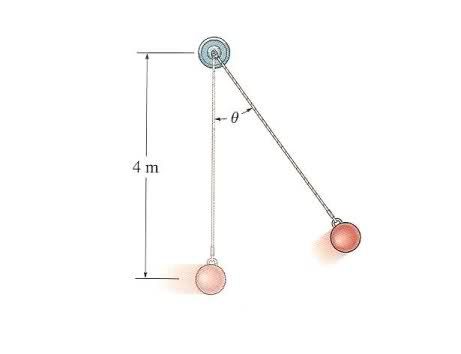# Determining tension

red123

## Homework Statement

The Ball has a mass of 30 kg and a speed v = 4 m/s at the instant it is at its lowest point, θ = 0°. Determine the tension in the cord and the rate at which the ball's speed is decreasing at the instant θ = 20°. Neglect the size of the ball.ΣF = ma
an = v2

## The Attempt at a Solution

T = mg + mv2
= 30kg(9.81 m/s2) + 30kg(4 m/s2)
= 414.3 N

Last edited:

## Answers and Replies

Homework Helper
That looks correct. So onto the second part.

Redsummers
I would check the velocity at the point θ = 20º, because as I'm seeing in your attempt you're using the same very velocity as θ = 0º.

red123
That looks correct. So onto the second part.

It doesn't agree with my textbook's answer, T = 361 N

Homework Helper
It doesn't agree with my textbook's answer, T = 361 N

If that was the case, then v≠4 m/s.

Redsummers
Well, yeah the thing is that v = 4m/s at θ = 0º; but it is clear that at θ = 20º the velocity should differ, which should be easily calculated by energy conservation.

Homework Helper
Well, yeah the thing is that v = 4m/s at θ = 0º; but it is clear that at θ = 20º the velocity should differ, which should be easily calculated by energy conservation.

If you use the tension given as the answer and work towards a velocity, it will be less than 4.

Redsummers
Yeah that's how it should be. For example:

$$E_t = mgh + 1/2mv^2$$ so, as the h increases according to the angle, the potential will also do so, while the velocity will drop.

benhou

## Homework Equations

an = v2

Your problem occurred right here. Note that the ball is not moving in uniform speed, it drops down before it completes a loop. Therefore, it is not uniform circular motion and this equation does not work over here. Also note that naturally, tension force does not exceed the bigger external force on the bodies. That means at the lowest point, the tension equals to the force of gravity.
Another way of thinking of it is optimization. A graph reaches it's maximum when the slope of the tangent is 0. In a v-t graph, the slopes of tangents are the accelerations of the specific instants. Since the slope of the tangent is 0 at the lowest point, therefore the acceleration is 0. This leads to F_net=0.

benhou
Try using conservation of energy.

Determine the tension in the cord and the rate at which the ball's speed is decreasing at the instant θ = 20°. Neglect the size of the ball.

Another problem you had was this. you are to find tension and acceleration at 20o